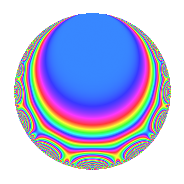# Properties

 Label 1224.1.nLevel $1224$ Weight $1$ Character orbit 1224.n Rep. character $\chi_{1224}(883,\cdot)$ Character field $\Q$ Dimension $5$ Newform subspaces $3$ Sturm bound $216$ Trace bound $2$

# Related objects

## Defining parameters

 Level: $$N$$ $$=$$ $$1224 = 2^{3} \cdot 3^{2} \cdot 17$$ Weight: $$k$$ $$=$$ $$1$$ Character orbit: $$[\chi]$$ $$=$$ 1224.n (of order $$2$$ and degree $$1$$) Character conductor: $$\operatorname{cond}(\chi)$$ $$=$$ $$136$$ Character field: $$\Q$$ Newform subspaces: $$3$$ Sturm bound: $$216$$ Trace bound: $$2$$

## Dimensions

The following table gives the dimensions of various subspaces of $$M_{1}(1224, [\chi])$$.

Total New Old
Modular forms 16 7 9
Cusp forms 8 5 3
Eisenstein series 8 2 6

The following table gives the dimensions of subspaces with specified projective image type.

$$D_n$$ $$A_4$$ $$S_4$$ $$A_5$$
Dimension 5 0 0 0

## Trace form

 $$5q + q^{2} + 5q^{4} + q^{8} + O(q^{10})$$ $$5q + q^{2} + 5q^{4} + q^{8} + 5q^{16} + q^{17} - 2q^{19} + 3q^{25} + q^{32} - 3q^{34} - 2q^{38} - 6q^{43} + 3q^{49} - q^{50} - 2q^{59} + 5q^{64} - 2q^{67} + q^{68} - 8q^{70} - 2q^{76} - 2q^{83} + 2q^{86} + 2q^{89} - q^{98} + O(q^{100})$$

## Decomposition of $$S_{1}^{\mathrm{new}}(1224, [\chi])$$ into newform subspaces

Label Dim. $$A$$ Field Image CM RM Traces $q$-expansion
$$a_2$$ $$a_3$$ $$a_5$$ $$a_7$$
1224.1.n.a $$1$$ $$0.611$$ $$\Q$$ $$D_{2}$$ $$\Q(\sqrt{-2})$$, $$\Q(\sqrt{-34})$$ $$\Q(\sqrt{17})$$ $$1$$ $$0$$ $$0$$ $$0$$ $$q+q^{2}+q^{4}+q^{8}+q^{16}+q^{17}-2q^{19}+\cdots$$
1224.1.n.b $$2$$ $$0.611$$ $$\Q(\sqrt{2})$$ $$D_{4}$$ $$\Q(\sqrt{-34})$$ None $$-2$$ $$0$$ $$0$$ $$0$$ $$q-q^{2}+q^{4}-\beta q^{5}-\beta q^{7}-q^{8}+\beta q^{10}+\cdots$$
1224.1.n.c $$2$$ $$0.611$$ $$\Q(\sqrt{2})$$ $$D_{4}$$ $$\Q(\sqrt{-34})$$ None $$2$$ $$0$$ $$0$$ $$0$$ $$q+q^{2}+q^{4}-\beta q^{5}+\beta q^{7}+q^{8}-\beta q^{10}+\cdots$$

## Decomposition of $$S_{1}^{\mathrm{old}}(1224, [\chi])$$ into lower level spaces

$$S_{1}^{\mathrm{old}}(1224, [\chi]) \cong$$ $$S_{1}^{\mathrm{new}}(136, [\chi])$$$$^{\oplus 3}$$

## Hecke characteristic polynomials

$p$ $F_p(T)$
$2$ ($$1 - T$$)($$( 1 + T )^{2}$$)($$( 1 - T )^{2}$$)
$3$ 1
$5$ ($$1 + T^{2}$$)($$1 + T^{4}$$)($$1 + T^{4}$$)
$7$ ($$1 + T^{2}$$)($$1 + T^{4}$$)($$1 + T^{4}$$)
$11$ ($$( 1 - T )( 1 + T )$$)($$( 1 - T )^{2}( 1 + T )^{2}$$)($$( 1 - T )^{2}( 1 + T )^{2}$$)
$13$ ($$( 1 - T )( 1 + T )$$)($$( 1 - T )^{2}( 1 + T )^{2}$$)($$( 1 - T )^{2}( 1 + T )^{2}$$)
$17$ ($$1 - T$$)($$( 1 - T )^{2}$$)($$( 1 + T )^{2}$$)
$19$ ($$( 1 + T )^{2}$$)($$( 1 + T^{2} )^{2}$$)($$( 1 + T^{2} )^{2}$$)
$23$ ($$1 + T^{2}$$)($$1 + T^{4}$$)($$1 + T^{4}$$)
$29$ ($$1 + T^{2}$$)($$1 + T^{4}$$)($$1 + T^{4}$$)
$31$ ($$1 + T^{2}$$)($$1 + T^{4}$$)($$1 + T^{4}$$)
$37$ ($$1 + T^{2}$$)($$1 + T^{4}$$)($$1 + T^{4}$$)
$41$ ($$( 1 - T )( 1 + T )$$)($$( 1 - T )^{2}( 1 + T )^{2}$$)($$( 1 - T )^{2}( 1 + T )^{2}$$)
$43$ ($$( 1 - T )^{2}$$)($$( 1 + T )^{4}$$)($$( 1 + T )^{4}$$)
$47$ ($$( 1 - T )( 1 + T )$$)($$( 1 - T )^{2}( 1 + T )^{2}$$)($$( 1 - T )^{2}( 1 + T )^{2}$$)
$53$ ($$( 1 - T )( 1 + T )$$)($$( 1 - T )^{2}( 1 + T )^{2}$$)($$( 1 - T )^{2}( 1 + T )^{2}$$)
$59$ ($$( 1 + T )^{2}$$)($$( 1 + T^{2} )^{2}$$)($$( 1 + T^{2} )^{2}$$)
$61$ ($$1 + T^{2}$$)($$1 + T^{4}$$)($$1 + T^{4}$$)
$67$ ($$( 1 + T )^{2}$$)($$( 1 + T^{2} )^{2}$$)($$( 1 + T^{2} )^{2}$$)
$71$ ($$1 + T^{2}$$)($$1 + T^{4}$$)($$1 + T^{4}$$)
$73$ ($$( 1 - T )( 1 + T )$$)($$( 1 - T )^{2}( 1 + T )^{2}$$)($$( 1 - T )^{2}( 1 + T )^{2}$$)
$79$ ($$1 + T^{2}$$)($$1 + T^{4}$$)($$1 + T^{4}$$)
$83$ ($$( 1 + T )^{2}$$)($$( 1 + T^{2} )^{2}$$)($$( 1 + T^{2} )^{2}$$)
$89$ ($$( 1 - T )^{2}$$)($$( 1 - T )^{4}$$)($$( 1 + T )^{4}$$)
$97$ ($$( 1 - T )( 1 + T )$$)($$( 1 - T )^{2}( 1 + T )^{2}$$)($$( 1 - T )^{2}( 1 + T )^{2}$$)